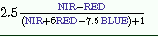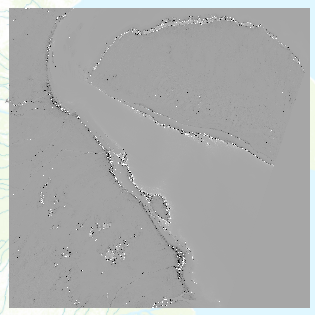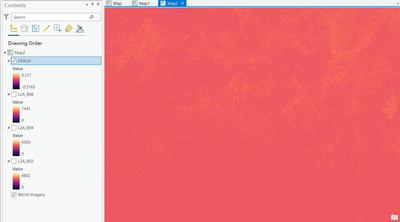# EVI calculation with Sentinel 2A data

804
2
09-03-2021 09:28 AM
Labels (2)by
New Contributor III

I'm using ArcGIS Pro 2.8.2. I've downloaded Sentinel 2A data, and would like to use raster calculator to calculate EVI. However, my results are outside of the -1 to 1 range, and the image it produces is very strange.

My code is:

``2.5*(("B08"-"B04")/(("B08"+6*"B04"-7.5*"B02")+1))``

where B02 is the blue band, B04 is the red band, and B08 is the NIR band. I based this code off of the general formula given here , which is:However, when I enter this, I get a range of -18405 to 18220, and my image looks like:Any advice on why this might be would be greatly appreciated.

Tags (3)
1 Solution

Accepted SolutionsbyMVP Esteemed Contributor

The formula considers the bands to be atmospherically corrected (L2A bands).

First you need to do an atmospheric correction using Sen2Cor (Check page No. 63, for the download option).

1. After download, extract the zip file in desired folder (no space in folderpath).

2. From Command Prompt, change directory to the extracted folder. Then execute a similar command as follows.

``L2A_Process.bat --resolution 10 <Input Raster Folder path till *.SAFE folder>``This process may take a while.

Next, Add the desired bands from the newly created *L2A* folder (R10m subfolder) to ArcGIS Pro. Use these bands for the calculation as follows.

``(2.5 * ("L2A_B08" - "L2A_B04") / (("L2A_B08" + 6.0 * "L2A_B04" - 7.5 * "L2A_B02") + 1.0))/10000``*My Area of Interest (here) is a desert with sparse vegetation.

Think Location
2 RepliesbyMVP Esteemed Contributor

When I have had this problem I have sampled band values of targeted vegetated and non-vegetated areas in the image and tested out the index in Excel or a calculator and if those are reasonable, then you know it is a software issue. A question that comes to mind is whether the index calculation is expecting an atmospheric or reflectance correction to be done before the index is calculated.byMVP Esteemed Contributor

The formula considers the bands to be atmospherically corrected (L2A bands).

First you need to do an atmospheric correction using Sen2Cor (Check page No. 63, for the download option).

1. After download, extract the zip file in desired folder (no space in folderpath).

2. From Command Prompt, change directory to the extracted folder. Then execute a similar command as follows.

``L2A_Process.bat --resolution 10 <Input Raster Folder path till *.SAFE folder>``This process may take a while.

Next, Add the desired bands from the newly created *L2A* folder (R10m subfolder) to ArcGIS Pro. Use these bands for the calculation as follows.

``(2.5 * ("L2A_B08" - "L2A_B04") / (("L2A_B08" + 6.0 * "L2A_B04" - 7.5 * "L2A_B02") + 1.0))/10000``*My Area of Interest (here) is a desert with sparse vegetation.

Think Location